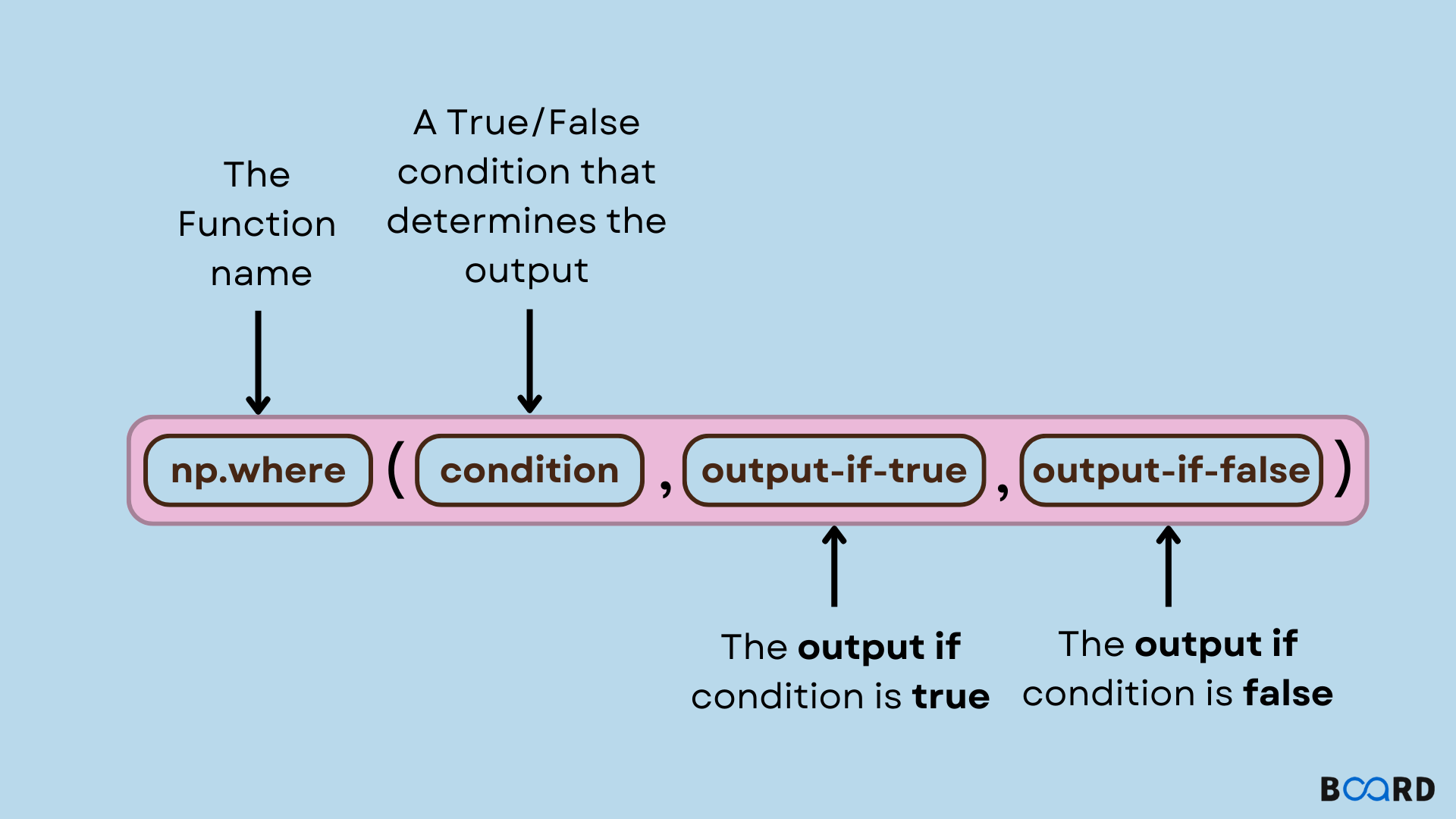Numpy Python

# numpy.where() in python## Introduction

NumPy is a Python library used for working with arrays.NumPy has various functions. Those functions are numpy array functions. One of the function is numpy.where(). It is used to return the indices of elements in an input array where the given condition is satisfied.

## Syntax

 numpy.where(condition, [x, y, ])Condition -  When True, yield x, otherwise yield y.x,y - Values from which to choose.

## Return Value of numpy.where()

1. If both x and y are specified then it returns elements of x where the condition is True and elements of y where the condition is false.
2. If only condition is given, then it returns the tuple of indices of element where condition is true

The exact same circumstance can also be shown as a = 2. Given how difficult it is to write the condition array as a boolean array, this is the format that is advised.

But what if we want to keep the result's dimension while retaining the items of our original array? Numpy.where() is a useful tool for this.

There are two additional variables: x and y. What do those mean?

In essence, this states that the new array will select elements from x if the condition is true for any element in our array.

If it is false, however, elements from y will be taken.

This will result in an array with items from x whenever condition is True and elements from y whenever condition is False in our final output array.

Although x and y are optional, it should be noted that if you specify x, you MUST also specify y. This is due to the requirement that the output array's shape match that of the input array in this situation.

## Examples

### Example 1

function with condition

 import numpy as nparr = np.array([[14,17,23,24,77,50,87]])arr1 = np.where(arr > 50) #conditionprint(arr1)

Output:

 (array([0, 0]), array([4, 6]))

Here the condition is arr>50. So the index of elements where value is greater than 50 is returned as tuple.

write your code here: Coding Playground

### Example 2

Condition with x and y value

 import numpy as nparr = np.array([[14,17,23,24,77,50,87]])arr2 = np.where(arr > 50, 1, 3) # x--> 1 , y-->3 print(arr2)

Output:

 [[3 3 3 3 1 3 1]]

Here condition along with x and y values are given which returns an array where condition satisfied index position is indicated by 1 and not satisfied condition with 3.

write your code here: Coding Playground

### Example 3

2D array

 import numpy as nparr = np.array([[12,14,17,19],[13,16,24,27]])  #2D arrayarr2 = np.where(arr <17, 1, 0)print(arr2)

Output:

 [[1 1 0 0] [1 1 0 0]]

Here each element of 2D array is checked for the condition and corresponding x and y value are returned.

write your code here: Coding Playground

### Example 4

numpy.where() without condition statement

 import numpy as nparr2 = np.where([True, False, False], [12,56,89], [13,12,90]) # X→[12,56,89] y→ [13,12,90]print(arr2)

Output:

 [12 12 90]

Here function returns array according to boolean condition. True will yield element from X array and False will yield element from Y array.

write your code here: Coding Playground

Numpy will broadcast every condition, x, and y array that we supply.

 import numpy as npa = np.arrange(12).reshape(3, 4)b = np.arrange(4).reshape(1, 4)print(a)print(b)# Broadcasts (a < 5, a, and b * 10)# of shape (3, 4), (3, 4) and (1, 4)c = np.where(a < 5, a, and b * 10)print(c)

Output:

 [[ 0  1  2  3] [ 4  5  6  7] [ 8  9 10 11]][[0 1 2 3]][[ 0  1  2  3] [ 4 10 20 30] [ 0 10 20 30]]
write your code here: Coding Playground

Again, the output is chosen based on the condition in this case, thus all elements are considered, but in this case, b is broadcast to the shape of a. (Since one of its dimensions only has one element, there won't be any mistakes when broadcasting.)

Therefore, b will now be [[0 1 2 3]]. We may now choose elements even from this broadcasted array [0 1 2 3] [0 1 2 3].

As a result, the output's shape matches that of a.